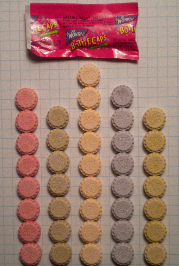Halloween night was rainy, so many fewer kids knocked on the door than usual. Consequently, I'm left with a big bucket of undistributed candy.

One evening as I treated myself to a mouthful of tooth decay, I chanced to open a package of Wonka® Bottle Caps. The package contained three little soda-flavored candies, and I was surprised to find that all three were the same flavor: grape. "Hey," I exclaimed to my youngest child, "they're all the same color! What do you think are the odds of that? You want to help me check some other packages?"

"Mom! Daddy's making me work on his blog," she complained.

After assuring her that she could devour the data when I finished my analysis, she helped me open the remaining packages of Bottle Caps and tabulate the flavors of the candies within.

The flavors of Bottle Caps candies are cola, root beer, grape, cherry, and orange. As we unwrapped the sugary data, my daughter and I hypothesized that the flavors of Bottle Caps were evenly distributed.

Although the hypothesis is the obvious one to make, it is not necessarily true for other candies. There have been dozens (hundreds?) of studies about the distribution of colors in M&M® candies. In fact, many math and statistics classes count the colors of M&M candies and compare the empirical counts to official percentages that are supplied by the manufacturer. The great thing about this classic project is that the colors of M&Ms are not uniformly distributed. Last time I checked, the official proportions were 30% brown, 20% yellow, 20% red, 10% orange, 10% green, and 10% blue.

So what about the Bottle Caps? There were 101 candies in 34 packages (one package contained only two candies). If our hypothesis is correct, the expected number of each flavor is 20.2 candies. We counted 23 Cherry, 15 Cola, 21 Grape, 25 Orange, and 17 Root Beer.

I entered the data into SAS for each package. (If you'd like to do further analysis, you can download the data.) Each package is a sample from a multinomial distribution, and I want to test whether the five flavors each have the same proportion, which is 1/5. I used the FREQ procedure to analyze the distribution of flavors and to run a chi-square test for equal proportions:

```proc freq data=BottleCaps; label Flavor = "Flavor"; weight Count; tables Flavor / nocum chisq plots=DeviationPlot; run;```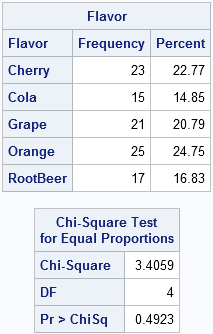The chi-square test gives a large p-value, so there is no statistical indication that the proportions of flavors of Bottle Caps are not uniform. All of the deviations can be attributed to random sampling.

The FREQ procedure can automatically produce plots as part of the analysis. For these data, I asked for a bar chart that shows the relative deviations of the observed frequencies (O) from the expected frequencies (E). The relative deviation is part of the chi-square computation, and is computed as (O-E)/E.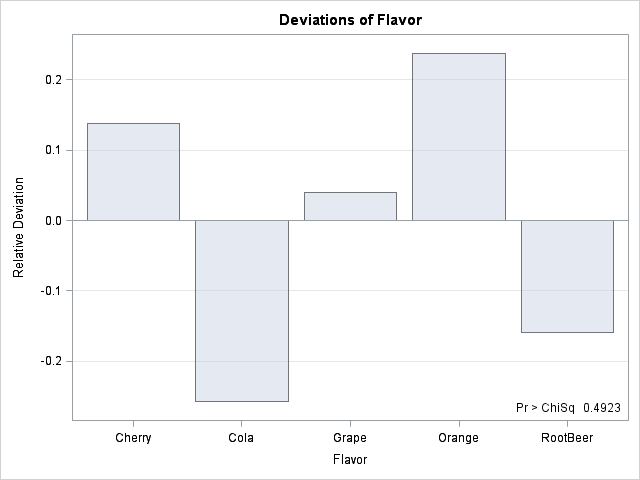Although the Bottle Caps that I observed had fewer cola and root beer flavors than expected, the chi-square test shows that these deviations are not significant. Anyway, my favorite Bottle Caps are cherry and orange, so I think the sampling gods smiled on me during this experiment.

Conclusion? Looks like Wonka makes the same number of each flavor. Now back to my pile of sugary goodness. I wonder how many candies are in a typical box of Nerds...Being able to reshape data is a useful skill in data analysis. Most of the time you can use the TRANSPOSE procedure or the SAS DATA step to reshape your data. But the SAS/IML language can be handy, too.

I only use PROC TRANSPOSE a few times per year, so my skills never progress beyond the "beginner" stage. I always have to look up the syntax! Sometimes, when I am trying to meet a deadline, I resort to using the SAS/IML language, which I find more intuitive for reshaping data.

Recently I had data that contained two variables: a character categorical variable and a numerical variable. I wanted to reshape the data so that each level (category) of the categorical variable became a new variable, and I wanted to use the levels to name the new variables. (This is an example of converting data from a "long" description to a "wide" description.) Because the number of observations usually differs among categories, some of the new variables will have missing values.

### A Canonical Example

A simple example is given by the Sashelp.Class data set. The SEX variable contains two values, F and M. There are several numerical variables; I'll use HEIGHT for this example. For these data, I want to reshape the data to create two variables named X_F and X_M, where the first variable contains the heights of the females and the second variable contains the heights of the males. The following DATA step shows one way to accomplish this:

```data combo; keep x_F x_M; merge sashelp.class(where=(sex="F") rename=(height=x_F)) sashelp.class(where=(sex="M") rename=(height=x_M)); run; proc print; run;```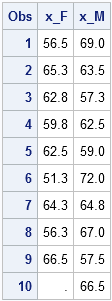Notice that the X_F variable contains a missing value because there are only nine females in the data, whereas there are ten males.

This technique works when you know the levels of the categorical variable, which you can discover by using PROC FREQ. However, what do you do if the categorical variable has dozens or hundreds of levels? It would be tedious to have to type in the generalization of this DATA step. I'd prefer to have code that works for an arbitrary categorical variable with k levels, and that automatically forms the names of the new variables as X_C1, X_C2, ..., X_Ck where C1, C2, ..., are the unique values of the categorical variable.

Obviously, this can be done, but I had a deadline to meet. Rather than mess with SAS macro, PROC SQL, and other tools that I do not use every day, I turned to the SAS/IML language

### SAS/IML to the Rescue

To reshape the data, I needed to do the following:

1. Find the levels (unique values) of the categorical variable and count the number of observations in each level.
2. Create the names of the new variables by appending the values of each level to the prefix "X_".
3. Allocate a matrix large enough to hold the results.
4. For the ith level of the categorical variable, copy the corresponding values from the continuous variable into the ith column of the matrix.

The following SAS/IML statements implement this algorithm:

```proc iml; use sashelp.class; read all var {sex} into C; read all var {height} into x; close;   /* TABULATE is SAS 9.3; you can also use UNIQUE + LOC */ call tabulate(u, count, C); /* 1. find unique values and count obs */ labels = "x_" + u; /* 2. create new variable names */   y = j(max(count), ncol(count), .); /* 3. allocate result matrix */ do i = 1 to ncol(count); /* 4. for each level... */ y[1:count[i], i] = x[loc(C=u[i])]; /* copy values into i_th column */ end; print y[colname=labels];```

The y matrix contains the desired values. Each column corresponds to a level of the categorical variable. Notice how the LOC function (also known as "the most useful function you've never heard of") is used to identify the observations for each category; the output from the LOC function is used to extract the corresponding values of x.

The program not only works for the Sashelp.Class data, it works in general. The TABULATE function (new in SAS 9.3) finds the unique values of the categorical variable and counts the number of observations for each value. If you do not have SAS 9.3, you can use the UNIQUE-LOC technique to obtain these values (see Section 3.3.5 of my book, Statistical Programming with SAS/IML Software). The remainder of the program is written in terms of these quantities. So, for example, if I want to reshape the MPG_CITY variable in the Sashelp.Cars data according to levels of the ORIGIN variable, all I have to do is change the first few lines of the program:

```use sashelp.cars; read all var {origin} into C; read all var {mpg_city} into x;```

Obviously, this could also be made into a macro or a SAS/IML module[REF], where the data set name, the name of the categorical variable, and the name of the numerical variable are included as parameters. It is also straightforward to support numerical categorical variables.

### Yes, it can be done with Base SAS...

Although the SAS/IML solution is short, simple, and does not require any macro shenanigans, I might as well provide a generalization of the earlier DATA step program. The trick is to use PROC SQL to compute the number of levels and the unique values of the categorical variable, and to put this information into SAS macro variables, like so:

```proc sql noprint; select strip(put(count(distinct sex),8.)) into :Count from sashelp.class; select distinct sex into :C1- :C&Count from sashelp.class; quit;```

The macro variable Count contains the number of levels, and the macro variables C1, C2,... contain the unique values. Using these quantities, you can write a short macro loop that generalizes the KEEP and MERGE statements in the original DATA step:

```/* create the string x_C1 x_C2 ... where C_i are unique values */ %macro keepvars; %do i=1 %to &Count; x_&&C&i %end; %mend;   /* create the various data sets to merge together, and create variable names x_C1 x_C2 ... where C_i are unique values */ %macro mergevars; %do i=1 %to &Count; sashelp.class(where=(sex="&&C&i") rename=(height=x_&&C&i)) %end; %mend;   data combo; keep %keepvars; merge %mergevars; run;```

Again, this could be made into a macro where the data set name, the name of the categorical variable, and the name of the numerical variable are included as parameters.

I'd like to thank my colleagues Jerry and Jason for ideas that led to the formulation of the preceding macro code. My colleagues also suggested several other methods for accomplishing the same task. I invite you to post your favorite technique in the comments.

Addendum (11:00am): Several people asked why I want to do this. The reason is that some procedures do not support a classification variable (or don't handle classification variables the way I want). By using this transformation, you can create multiple variables and have a procedure operate on those. For example, the DENSITY statement in PROC SGPLOT does not support a GROUP= option, but you can use this trick to overlay the densities of subgroups.

In reponse to this post, several other techniques in Base SAS were submitted to the SAS-L discussion forum. I particularly like Nat Wooding's solution, which uses PROC TRANSPOSE."I think that my data are exponentially distributed, but how can I check?"

I get asked that question a lot. Well, not specifically that question. Sometimes the question is about the normal, lognormal, or gamma distribution. A related question is "Which distribution does my data have," which was recently discussed by John D. Cook on his blog.

Regardless of the exact phrasing, the questioner wants to know "What methods are available for checking whether a given distribution fits the data?" In SAS, I recommend the UNIVARIATE procedure. It supports three techniques that are useful for comparing the distribution of data to some common distributions: goodness-of-fit tests, overlaying a curve on a histogram of the data, and the quantile-quantile (Q-Q) plot. (Some people drop the hyphen and write "the QQ plot.")

### Constructing a Q-Q Plot for any distribution

The UNIVARIATE procedure supports many common distributions, such as the normal, exponential, and gamma distributions. In SAS 9.3, the UNIVARIATE procedure supports five new distributions. They are the Gumbel distribution, the inverse Gaussian (Wald) distribution, the generalized Pareto distribution, the power function distribution, and the Rayleigh distribution.

But what if you want to check whether your data fits some distribution that is not supported by PROC UNIVARIATE? No worries, creating a Q-Q plot is easy, provided you can compute the quantile function of the theoretical distribution. The steps are as follows:

1. Sort the data.
2. Compute n evenly spaced points in the interval (0,1), where n is the number of data points in your sample.
3. Compute the quantiles (inverse CDF) of the evenly spaced points.
4. Create a scatter plot of the sorted data versus the quantiles computed in Step 3.

If the data are in a SAS/IML vector, the following statements carry out these steps:

```proc iml; y = {1.7, 1.0, 0.5, 3.5, 1.9, 0.7, 0.4, 5.1, 0.2, 5.6, 4.6, 2.8, 3.8, 1.4, 1.6, 0.9, 0.3, 0.4, 1.9, 0.5};   n = nrow(y); call sort(y, 1); /* 1 */ v = ((1:n) - 0.375) / (n + 0.25); /* 2 (Blom, 1958) */ q = quantile("Exponential", v, 2); /* 3 */```

If you plot the data (y) against the quantiles of the exponential distribution (q), you get the following plot: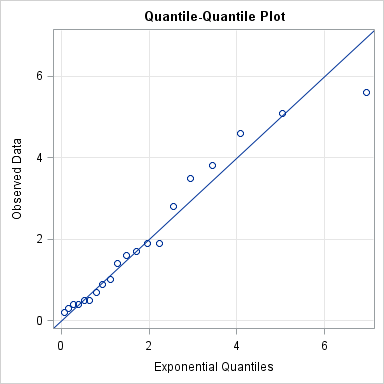"But, Rick," you might argue, "the plotted points fall neatly along the diagonal line only because you somehow knew to use a scale parameter of 2 in Step 3. What if I don't know what parameter to use?!"

Ahh, but that is the beauty of the Q-Q plot! If you plot the data against the standardized distribution (that is, use a unit scale parameter), then the slope of the line in a Q-Q plot is an estimate of the unknown scale parameter for your data! For example, modify the previous SAS/IML statements so that the quantiles of the exponential distribution are computed as follows: `q = quantile("Exponential", v); /* 3 */`

The resulting Q-Q plot shows points that lie along a line with slope 2, which implies that the distribution of the data is approximately exponentially distributed with a shape parameter close to 2.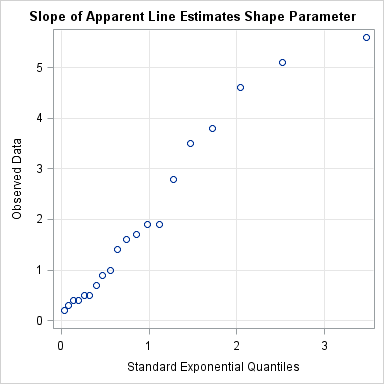### Choice of quantiles for the theoretical distribution

The Wikipedia article on Q-Q plots states, "The choice of quantiles from a theoretical distribution has occasioned much discussion." Wow, is that an understatement! Literally dozens of papers have been written on this topic. SAS uses a formula suggested by Blom (1958): (i - 3/8) / (n + 1/4), i=1,2,...,n. Another popular choice is (i-0.5)/n, or even i/(n+1). For large n, the choices are practically equivalent. See O. Thas (2010), Comparing Distributions, p. 57–59 for a discussion of various choices. In SAS, you can use the RANKADJ= and NADJ= options to accomodate different choices.

### Repeating the construction by using the DATA step

These computations are simple enough to perform by using the DATA step and PROC SORT. For completeness, here is the SAS code:

```data A; input y @@; datalines; 1.7 1.0 0.5 3.5 1.9 0.7 0.4 5.1 0.2 5.6 4.6 2.8 3.8 1.4 1.6 0.9 0.3 0.4 1.9 0.5 ; run; proc sort data=A; by y; run; /* 1 */ data Exp; set A nobs=nobs; v = (_N_ - 0.375) / (nobs + 0.25); /* 2 */ q = quantile("Exponential", v, 2); /* 3 */ run;   proc sgplot data=Exp noautolegend; /* 4 */ scatter x=q y=y; lineparm x=0 y=0 slope=1; /* SAS 9.3 statement */ xaxis label="Exponential Quantiles" grid; yaxis label="Observed Data" grid; run;```

### Use PROC UNIVARIATE for Simple Q-Q Plots

Of course, for this example, I don't need to do any computations at all, since PROC UNIVARIATE supports the exponential distribution and other common distributions. The following statements compute goodness-of-fit tests, overlay a curve on the histogram, and display a Q-Q plot:

```proc univariate data=A; var y; histogram y / exp(sigma=2); QQplot y / exp(theta=0 sigma=2); run;```

However, if you think your data are distributed according to some distribution that is not built into PROC UNIVARIATE, the techniques in this article show how to construct a Q-Q plot to help you assess whether some "named" distribution might model your data.When I learn a new statistical technique, one of first things I do is to understand the limitations of the technique. This blog post shares some thoughts on modeling finite mixture models with the FMM procedure. What is a reasonable task for FMM? When are you asking too much?

I previously showed how you can use the FMM procedure to model Scrabble® scores as a mixture of three components: typical plays, triple-word scores, and penalties. That experience taught me three lessons about finite mixture models and their ability to "resolve" components that are close to each other:

• The further away the components are, the easier it is to resolve them.
• The more data you have, the better you can resolve components.
• If you can give the software hints about the components, you can resolve them better.

These lessons are not surprising, and the first two are used to determine the "power" of a statistical test. In brief, the larger the "effect" you are trying to measure, the easier it is to measure it. Similarly, larger samples have less sampling error than smaller samples, so an effect is easier to detect in larger samples. The third statement (give hints) is familiar to anyone who has used maximum likelihood estimation: a good guess for the parameter estimates can help the algorithm converge to an optimal value.

To be concrete, suppose that a population is composed of a mixture of two normal densities. Given a sample from the population, can the FMM procedure estimate the mean and variance of the underlying components? The answer depends on how far apart the two means are (relative to their variances) and how large the sample is.

### The distance between components

The following figure shows the exact density for a 50/50 mixture of normal distributions, where the first component is N(0,1) and the second component is N(δ, 1). The figure shows two cases: δ=1 and 2.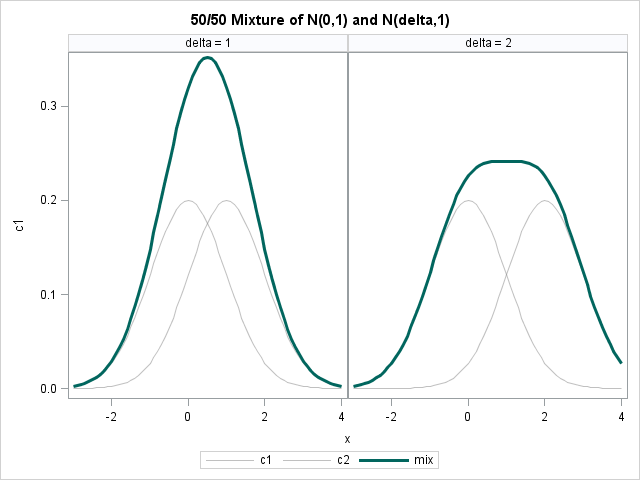Would a human be able to look at the dark curve on the left and deduce that it is a mixture of two normal components? Unlikely. The problem is that the distance between the component densities, which is 1, is not sufficiently large relative to the variances of the components (also 1). What about the dark curve on the right? Perhaps. It depends on the person's experience with mixture models.

What happens if you generate a small number of random values (say, 100) from each probability distribution, and ask the FMM procedure to try to detect two normal components in the data? The following statements generate random values from a mixture of normals with δ=1 and δ=2, and use the FMM procedure to try to estimate the two components:

```%let p = 0.5; %let n = 100; /* generate random values from mixture distribution */ data Sim(drop=i); call streaminit(12345); do delta = 1 to 2; do i = 1 to &n; c = rand("Bernoulli", &p); if c=0 then x = rand("normal"); else x = rand("normal", delta); output; end; end; run;   /* Doesn't identify underlying components for delta=1. But DOES for delta=2! */ proc fmm data=Sim; by delta; model x= / k=2; run;```

With only 100 points in the sample, the FMM procedure can't correctly identify the N(0,1) and N(1,1) components in the data for δ=1. However, it does correctly identify the components that are separated by a larger amount, as shown below: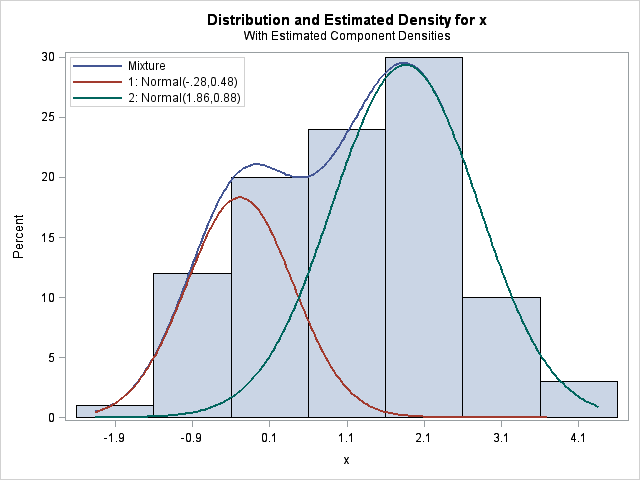The conclusions are clear, if you have a small sample, don't expect to the FMM procedure to be able to estimate components that practically overlap.

### More data = Better estimates

If you have a larger sample, the FMM procedure has more data to work with, and can detect components that do not appear distinct to the human eye. For example, if you rerun the previous example with, say, 10,000 simulated observations, then the FMM procedure has no trouble correctly identifying the N(0,1) and N(1,1) components.

This "lesson" (more data leads to better estimates) is as old as statistics itself. Yet, when applied to the FMM procedure, you can get some pretty impressive results, such as those described in Example 37.2 in the documentation for the FMM procedure. In this example (reproduced below), two normal components and a Weibull component are identified in data that does not—to the untrained eye—look like a mixture of normals. From biological arguments, researchers knew that the density should have three components. Because the biologists had a large number of observations (141,414 of them!), the FMM procedure is able to estimate the components.### More hints = Better estimates

"Well, great," you might say, "but my sample is small and I can't collect more data. Is there any other way to coax the FMM procedure into finding relatively small effects?"

Yes, you can provide hints to the procedure. The sample and the choice of a model determines a likelihood function, which the procedure uses to estimate the parameters in the model for which the data are most likely. The likelihood function might have several local maxima, and we can use the PARMS option in the MODEL statement to provide guesses for the parameters. I used this technique to help PROC FMM estimate parameters for the three-component model of my mother's Scrabble scores.

There is another important way to give a hint: you can tell the FMM procedure that one or more observations belong to particular components by using the PARTIAL= option in the PROC FMM statement. For example, it is difficult for the FMM procedure to estimate three normal components for the PetalWidth variable in the famous Fisher's iris data. Why? Because there are only 150 observations, and the means of two components are close to each other (relative to their variance). But for these data, we actually have a classification variable, Species, that identifies to which component each observation belongs. By using this extra information, the FMM procedure correctly estimates the three components:

```/* give partial information about component membership */ proc fmm data=sashelp.iris partial=Species; class Species; model PetalWidth = / K=3; run;```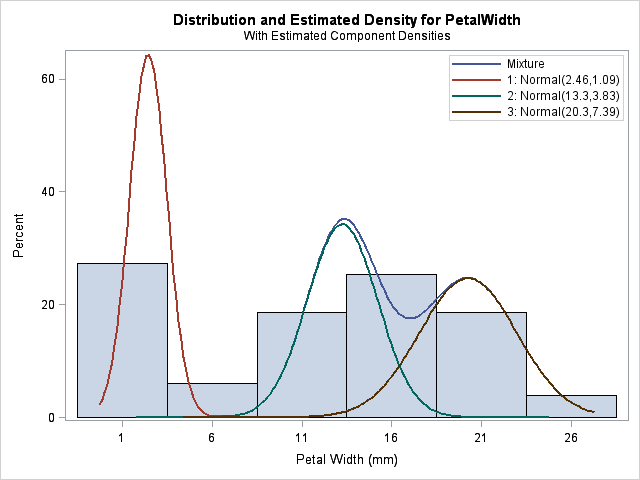Is this cheating? In this case, yes, because having completely labeled data is equivalent to doing three separate density estimates, one for each species. However, the PARTIAL= option is intended to be used when you have only partial information (hence its name) about which observations are members of which component. For example, the procedure can still estimate the three components when half of the Species variable is randomly set to missing:

```data iris; set sashelp.iris; if ranuni(1)<0.5 then Species=" "; /* set to missing */ run;```

It is important to understand the limitations of any statistical modeling procedure that you use. Modeling finite mixtures with PROC FMM is no exception. If you know which tasks are reasonable and which are unrealistic, you can be more successful as a data analyst.A popular use of SAS/IML software is to optimize functions of several variables. One statistical application of optimization is estimating parameters that optimize the maximum likelihood function. This post gives a simple example for maximum likelihood estimation (MLE): fitting a parametric density estimate to data.

### Which density curve fits the data?

If you plot a histogram for the SepalWidth variable in the famous Fisher's iris data, the data look normally distributed. The normal distribution has two parameters: μ is the mean and σ is the standard deviation. For each possible choice of (μ, σ), you can ask the question, "If the true population is N(μ, σ), how likely is it that I would get the SepalWidth sample?" Maximum likelihood estimation is a technique that enables you to estimate the "most likely" parameters. This is commonly referred to as fitting a parametric density estimate to data.

Visually, you can think of overlaying a bunch of normal curves on the histogram and choosing the parameters for the best-fitting curve. For example, the following graph shows four normal curves overlaid on the histogram of the SepalWidth variable:

```proc sgplot data=Sashelp.Iris; histogram SepalWidth; density SepalWidth / type=normal(mu=35 sigma=5.5); density SepalWidth / type=normal(mu=32.6 sigma=4.2); density SepalWidth / type=normal(mu=30.1 sigma=3.8); density SepalWidth / type=normal(mu=30.5 sigma=4.3); run;```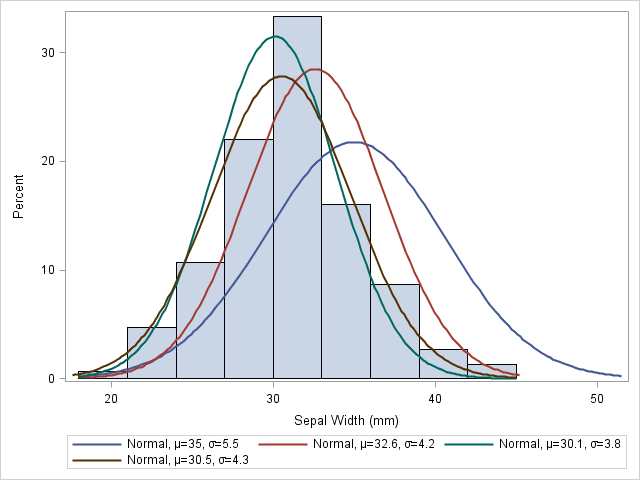It is clear that the first curve, N(35, 5.5), does not fit the data as well as the other three. The second curve, N(32.6, 4.2), fits a little better, but mu=32.6 seems too large. The remaining curves fit the data better, but it is hard to determine which is the best fit. If I had to guess, I'd choose the brown curve, N(30.5, 4.3), as the best fit among the four.

The method of maximum likelihood provides an algorithm for choosing the best set of parameters: choose the parameters that maximize the likelihood function for the data. Parameters that maximize the log-likelihood also maximize the likelihood function (because the log function is monotone increasing), and it turns out that the log-likelihood is easier to work with. (For the normal distribution, you can explicitly solve the likelihood equations for the normal distribution. This provides an analytical solution against which to check the numerical solution.)

### The likelihood and log-likelihood functions

The UNIVARIATE procedure uses maximum likelihood estimation to fit parametric distributions to data. The UNIVARIATE procedure supports fitting about a dozen common distributions, but you can use SAS/IML software to fit any parametric density to data. There are three steps:

1. Write a SAS/IML module that computes the log-likelihood function. Optionally, since many numerical optimization techniques use derivative information, you can provide a module for the gradient of the log-likelihood function. If you do not supply a gradient module, SAS/IML software automatically uses finite differences to approximate the derivatives.
2. Set up any constraints for the parameters. For example, the scale parameters for many distributions are restricted to be positive values.
3. Call one of the SAS/IML nonlinear optimization routines. For this example, I call the NLPNRA subroutine, which uses a Newton-Raphson algorithm to optimize the log-likelihood.

#### Step 1: Write a module that computes the log-likelihood

A general discussion of log-likelihood functions, including formulas for some common distributions, is available in the documentation for the GENMOD procedure. The following module computes the log-likelihood for the normal distribution:

```proc iml; /* write the log-likelihood function for Normal dist */ start LogLik(param) global (x); mu = param; sigma2 = param##2; n = nrow(x); c = x - mu; f = -n/2*log(sigma2) - 1/2/sigma2*sum(c##2); return ( f ); finish;```

Notice that the arguments to this module are the two parameters mu and sigma. The data vector (which is constant during the optimization) is specified as the global variable x. When you use an optimization method, SAS/IML software requires that the arguments to the function be the quantities to optimize. Pass in other parameters by using the GLOBAL parameter list.

It's always a good idea to test your module. Remember those four curves that were overlaid on the histogram of the SepalWidth data? Let's evaluate the log-likelihood function at those same parameters. We expect that log-likelihood should be low for parameter values that do not fit the data well, and high for parameter values that do fit the data.

```use Sashelp.Iris; read all var {SepalWidth} into x; close Sashelp.Iris;   /* optional: test the module */ params = {35 5.5, 32.6 4.2, 30.1 3.8, 30.5 4.3}; LogLik = j(nrow(params),1); do i = 1 to nrow(params); p = params[i,]; LogLik[i] = LogLik(p); end; print Params[c={"Mu" "Sigma"} label=""] LogLik;```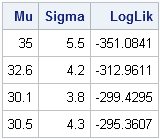The log-likelihood values confirm our expectations. A normal density curve with parameters (35, 5.5) does not fit the data as well as the other parameters, and the curve with parameters (30.5, 4.3) fits the curve the best because its log-likelihood is largest.

#### Step 2: Set up constraints

The SAS/IML User's Guide describes how to specify constraints for nonlinear optimization. For this problem, specify a matrix with two rows and k columns, where k is the number of parameters in the problem. For this example, k=2.

• The first row of the matrix specifies the lower bounds on the parameters. Use a missing value (.) if the parameter is not bounded from below.
• The second row of the matrix specifies the upper bounds on the parameters. Use a missing value (.) if the parameter is not bounded from above.

The only constraint on the parameters for the normal distribution is that sigma is positive. Therefore, the following statement specifies the constraint matrix:

```/* mu-sigma constraint matrix */ con = { . 0, /* lower bounds: -infty < mu; 0 < sigma */ . .}; /* upper bounds: mu < infty; sigma < infty */```

#### Step 3: Call an optimization routine

You can now call an optimization routine to find the MLE estimate for the data. You need to provide an initial guess to the optimization routine, and you need to tell it whether you are finding a maximum or a minimum. There are other options that you can specify, such as how much printed output you want.

```p = {35 5.5};/* initial guess for solution */ opt = {1, /* find maximum of function */ 4}; /* print a LOT of output */ call nlpnra(rc, result, "LogLik", p, opt, con);```

The following table and graph summarize the optimization process. Starting from the initial condition, the Newton-Raphson algorithm takes six steps before it reached a maximum of the log-likelihood function. (The exact numbers might vary in different SAS releases.) At each step of the process, the log-likelihood function increases. The process stops when the log-likelihood stops increasing, or when the gradient of the log-likelihood is zero, which indicates a maximum of the function.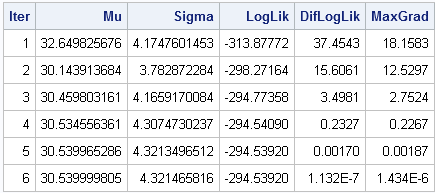You can summarize the process graphically by plotting the path of the optimization on a contour plot of the log-likelihood function. You can see that the optimization travels "uphill" until it reaches a maximum.### Check the optimization results

As I mentioned earlier, you can explicitly solve for the parameters that maximize the likelihood equations for the normal distribution. The optimal value of the mu parameter is the sample mean of the data. The optimal value of the sigma parameter is the unadjusted standard deviation of the sample. The following statements compute these quantities for the SepalWidth data:

```OptMu = x[:]; OptSigma = sqrt( ssq(x-OptMu)/nrow(x) );```

The values found by the NLPNRA subroutine agree with these exact values through seven decimal places.When you misspell a word on your mobile device or in a word-processing program, the software might "autocorrect" your mistake. This can lead to some funny mistakes, such as the following:

I hate Twitter's autocorrect, although changing "extreme couponing" to "extreme coupling" did make THAT tweet more interesting. [@AnnMariaStat]

When you type "hte," how does the software know that you probably meant "the"? Even more impressively, when you type "satitsisc" into Microsoft Word, how does it create a list of the words you might have meant, a list that includes "statistics"?

For that matter, have you every mistyped the name of a SAS procedure or an option, only to discover that your program still ran? For example, if you type dat a; run; the program will run, although the SAS log will warn you of the misspelling: "WARNING 14-169: Assuming the symbol DATA was misspelled as dat." How can SAS determine that what you typed is close enough to an actual keyword that it assumes that the keyword was misspelled?

There are various techniques for correcting misspellings, but most of them involve a notion of the distance between words. Given a string of letters, you can define a number that relates how "close" the string is to other words (for example, in a dictionary). The string that is typed is called the keyword. The words in the dictionary are called query words. If the keyword is not in the dictionary, then you can list the query words that are close to the keyword. Often the keyword is a misspelling of one of the words on this list.

Base SAS has several functions that you can use to compare how close words are to each other. In this post, I describe the SPEDIS function, which I assume is an acronym for "spelling distance." Similar SAS functions include the COMPLEV function, the COMPGED function, and the SOUNDEX function.

### What words are close to "satitsisc"

The SPEDIS function computes the "cost" of converting the keyword to a query word. Each operation—deletion, insertion, transposition, and so forth—is assigned a certain cost.

As an example, type the letters "satitsisc" into Microsoft Word and look at the top words that Word suggests for the misspelling. The words are statistics, statistic, and sadistic. The words satisfy and Saturday are not on the list of suggested words. By using the SAS DATA step or the SAS/IML language, you can examine how close each of these words are to the garbled keyword, as measured by the SPEDIS function:

```proc iml; keyword = "satitsisc"; query = {"statistics" "statistic" "sadistic" "satisfy" "Saturday"}; cost = spedis(query, keyword); print cost;```According to the SPEDIS function, the words suggested by MS Word (the first three query words) have a lower cost than satisfy and Saturday, which were not suggested.

The cost returned by the SPEDIS function is not symmetric. For example, the cost of converting sadistic to statistic is different from the cost of converting statistic to sadistic, because inverse operations have different costs. For example, the cost of inserting a letter is different from the cost of deleting a letter.

This lack of symmetry means that the SPEDIS function does not return a true distance between words, although some people refer to the cost matrix as an "asymmetric distance." You can use the SAS/IML language to compute the cost matrix, in which the ijth element is the cost of converting the ith word into the jth word:

```words = query || keyword; n = ncol(words); /* compute (n x n) cost matrix (not symmetric) */ cost = j(n,n,0); do i = 1 to ncol(words); key = words[i]; cost[i,] = spedis(words, key); end; print cost[c=words r=words];```It is possible to visualize the distance between words by using the MDS procedure to compute how to position the words in the plane so that the planar distances most nearly match the (scaled) values in the cost matrix:

```/* write SAS/IML cost matrix to data set */ create cost(type=DISTANCE) from cost[c=words r=words]; append from cost[r=words]; close; quit;   /* find 2D configuration of points */ ods graphics on; proc mds data=cost dimension=2 shape=square; subject words; run;```PROC MDS creates a graph that is similar to the one shown. Notice that the cluster in the upper right consists of the keyword and the words that MS Word suggests. These words are close to the keyword. On the other hand, the two words that were not suggested by MS Word are situated far from the keyword.

This post was inspired by Charlie Huang's interesting article titled Top 10 most powerful functions for PROC SQL. The SPEDIS and SOUNDEX functions are listed in item #6.

If you want to read funny examples of mistakes in text messages that were caused by the autocorrect feature of the iPhone, do an internet search for "funny iPhone autocorrect mistakes." Be aware, however, that many of the examples involve adult humor.

You can use the SPEDIS function to do fuzzy matching, as shown in the following papers:In my previous post, I blogged about how to sample from a finite mixture distribution. I showed how to simulate variables from populations that are composed of two or more subpopulations. Modeling a response variable as a mixture distribution is an active area of statistics, as judged by many talks on the topic at JSM 2011. When SAS 9.3 shipped, I promised to discuss my experiences with the new experimental FMM procedure, which fits finite mixture models. This article describes modeling univariate data as a mixture of normal distributions.

### Initial thoughts and misconceptions

I confess: I don't know much about finite mixture models. I read some of the documentation and followed some of the examples, but I've barely begun to explore the capabilities of PROC FMM. (Thanks to my colleagues, Dave and John, for reviewing this article.)

The FMM procedure in SAS/STAT software is not a discrimination procedure. When you use the CANDISC and DISCRIM procedures, you must know the group membership for each observation in order to build a model that classifies the group membership of future observations. Similarly, most SAS regression procedures enable you to use a CLASS statement to build a model that accounts for group membership. The beauty of the FMM procedure is that you don't need this knowledge, and yet you can still build a model for response variables that are multi-modal. (You can, however, supply partial group information when you know it by using the PARTIAL option.) In my mind, I pretend that PROC FMM models the components as latent variables, since I'm familiar with that concept.

The way that the FMM procedure models a response variable reminds me of clustering procedures: You tell the FMM procedure that the response variable consists of k components, and the procedure tries to use the data to discover (and model) the group structure as part of building a regression model. This means that there are many parameters in this problem: there are the usual parameters for the regression effects, but there are also parameters that estimate the location, shape, and strength of each component.

### A first example: Modeling the distribution of Scrabble® scores

As a first example, I want to see whether the FMM procedure can model a univariate distribution as a mixture of normal densities. The data are the scores for each word played in series of a Scrabble games between my mother and me.

As I wrote previously, the distribution of scores seems to have three components. Most scores are in the 12–15 point range, and correspond to standard plays. There is a small group of larger scores that correspond to triple word scores. For my mother's scores, there is a third group of small and negative scores that correspond to plays made at the end of a game. You can download the data for these Scrabble games.

For the games recorded here, my scores had few end-of-game penalties. Consequently, I assume that my scores fall into two groups. I use the FMM procedure with the K=2 option to model my scores as a mixture of two normal distributions. Notice that I specify the response variable in the MODEL statement, but do not specify any covariate effects, so this is a model for the mean of the SCORE variable:

```proc fmm data=scrabble(where=(Player=2)); /* Rick's scores */ model Score = / k=2; run;```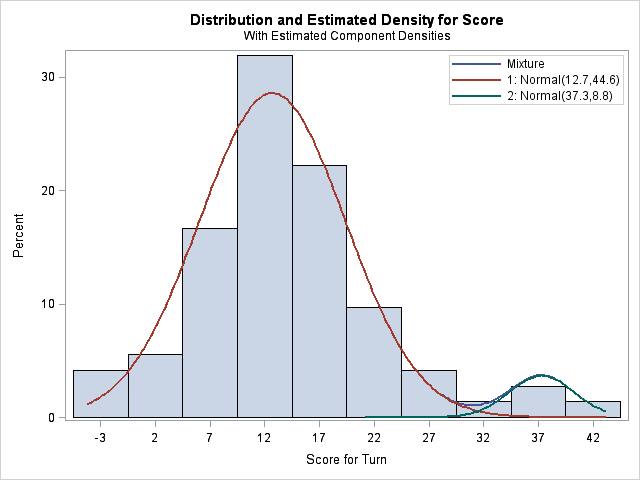The analysis models the distribution of scores as a mixture of two normal densities. The first component has mean 12.7, which represents my average score on a "typical" word play. The variance of this distribution is 44.6, which corresponds to a standard deviation of 6.7 points per play. The second component has a mean of 37.3 and a variance of 8.8 (a standard deviation just under 3 points), and represents my triple word score plays.

The blue curve, which is almost not visible, is the overall density estimate. It is a weighted sum of the two component densities. The weights sum to unity, so there is one parameter, called the mixing parameter, that needs to be estimated by the FMM procedure in addition to the two location and scale parameters for each component. For these data, the mixing probability estimate is 2.86. This estimate is on a logit scale, which means it corresponds to a mixing probability of exp(2.86)/(exp(2.86) +exp(0)) = 0.946. This number is shown in the "Probability" column of the second table. Equivalently, the estimated PDF is 0.95*f1 + 0.05*f2, where f1 is the PDF for the first component and f2 is the PDF for the second component. These weights make sense because my mother always "steals" the triple word plays from me, which means that I have few opportunities to play a triple word score!

Now I'll try to estimate my mother's scores as a mixture of three normal densities by using the following call to the FMM procedure:

```proc fmm data=scrabble(where=(Player=1)); /* Mom's scores */ model Score = / k=3; run;```

Unfortunately, this approach doesn't work very well for my mother's data. The FMM procedure converges to a set of parameters that correspond to a local maximum of the likelihood function. This solution models the data as a mixture of three distributions that are practically identical in terms of location and scale.

Fortunately, you can give "hints" to the FMM procedure by specifying starting values for the parameter estimates. The following call specifies a guess for the location and scale parameters, based on my knowledge of the game of Scrabble:

```proc fmm data=scrabble(where=(Player=1)); model Score = / k=3 parameters(-5 4, 13 49, 33 9); /* means: -5, 13, 33 */ run;```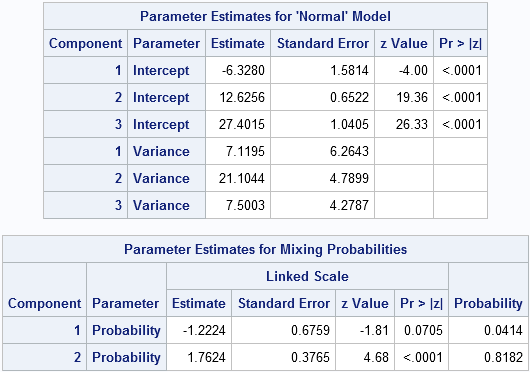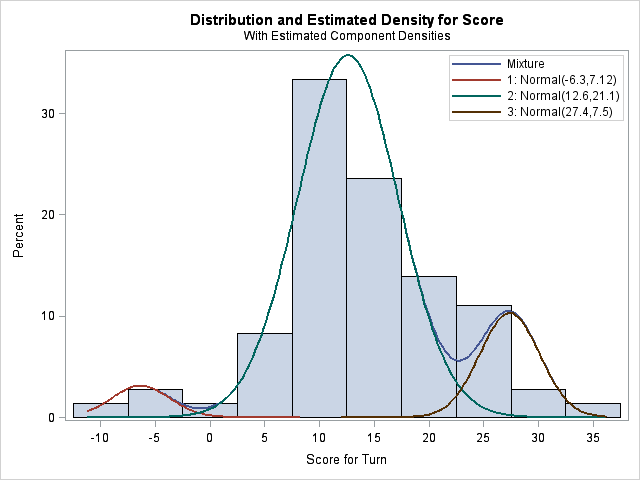This time, the FMM procedure converges to a model that satisfies my prior beliefs. The means of the three components are at -6.3, 12.6, and 27.4. The mixing probability estimates are shown in the "Probabilities" column. They are 0.04 and 0.82, which means that the third mixing probability estimate is 0.14. Notice that the triple-word-score component for my mother accounts for 14% of the probability, whereas it accounts for only 5% of my scores.

### Conclusions and gotchas

The FMM procedure did a good job of finding intuitive components for Scrabble scores. For my scores, it found two normal components such that the density of scores is a weighted sum of the components. For my mother's scores, it found three components, although I had to supply a hint. Notice that the procedure needs to estimate eight parameters in order to solve the three-component problem!

There were three lessons I learned from this exercise. The first two are "gotchas" regarding the 9.3 syntax:

• Unlike some other SAS functions, the FMM procedure represents scale in terms of the variance. If you are used to specifying the scale parameter as a standard deviation in the RAND, PDF, and CDF functions and in the UNIVARIATE and SGPLOT procedures, this convention might cause momentary confusion.
• Unlike the HISTOGRAM statement in the UNIVARIATE procedure, in which you specify all of the means followed by all of the standard deviations, the syntax of the PARAMETERS statement in the FMM procedure requires that you specify the mean and variance of each component in sequence.

The third lesson concerns the limitations of the FMM procedure. If two components of the response distribution are close to each other, then it is unreasonable to expect the FMM procedure to be able to model them as separate components. This is similar to the fact that clustering procedures have difficulties finding poorly separated clusters. I'll discuss this issue further in a future article.

tags: Data AnalysisLast week I showed a graph of the number of US births for each day in 2002, which shows a strong day-of-the-week effect. The graph also shows that the number of births on a given day is affected by US holidays. This blog post looks closer at the holiday effect. I actually conducted this analysis in 2009 for my book, but decided not to include it.

I want to identify days in 2002 that have fewer births than would be expected, given the day of the week. A box plot is often used for this sort of exploratory data analysis. The following statements use the VBOX statement in the SGPLOT procedure to create a box plot for each day of the week and to label the outliers for each day:

```proc sgplot data=birthdays2002; title "US Births by Day of Week (2002)"; vbox Percentage / category=Day datalabel=Date; yaxis grid; xaxis display=(nolabel) discreteorder=data; run;```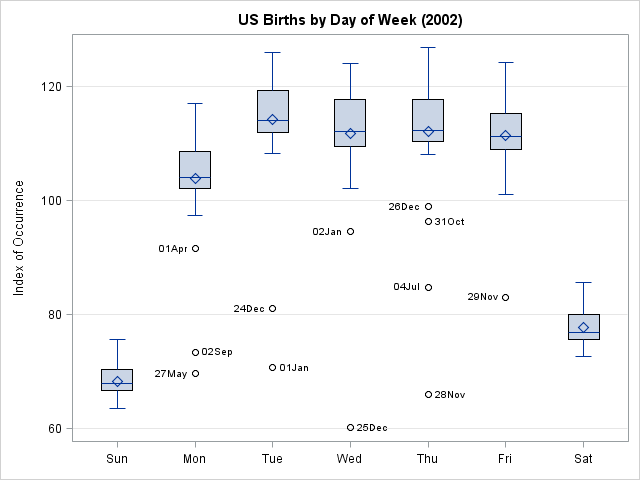In the box plots (click to enlarge), the outliers for each day of the week are labeled by using values of the Date variable. Each date belongs to one of the following categories: US holidays, days near holidays, and inauspicious days.

### US holidays

Several US holidays in 2002 are responsible for lower than expected births, given the day of the week:

• Tuesday, 01JAN (New Year's Day)
• Monday, 27MAY (Memorial Day)
• Thursday, 04JUL (Independence Day)
• Monday, 02SEP (Labor Day)
• Thursday, 28NOV (Thanksgiving Day)
• Wednesday, 25DEC (Christmas Day)

Christmas Day is the day on which the fewest babies were born.

Several "minor" holidays on Mondays also exhibit slightly smaller-than-expected births. These are not visible in the box plot graph, but can be seen in the time series graph: 21JAN (Birthday of Martin Luther King, Jr.), 18FEB (Washington's Birthday, sometimes known as "President's Day"), 14OCT (Columbus Day), and 11NOV (Veterans Day).

### Days near holidays

Families often travel on days near holidays, and that includes doctors and other hospital staff. Several of these days are visible as outliers in the birth data.

• Wednesday, 02JAN (day after New Year's Day)
• Friday, 29NOV (day after Thanksgiving Day)
• Tuesday, 24DEC (Christmas Eve)
• Thursday, 26DEC (day after Christmas Day)

Friday, 03JUL (the day prior to Independence Day), also exhibits smaller-than-expected births, as seen in the time series graph.

### Inauspicious days

The following dates are also outliers:

• Monday, 01APR (April Fool's Day)
• Thursday, 31OCT (Halloween Day)

Most parents don't want their child to be teased for being an "April Fool" all his life. It is less clear why a couple would avoid giving birth on Halloween. Superstition? Maybe. Or maybe doctors don't induce deliveries on Halloween so that they can be home for trick-or-treating?

These days might not be preferred for giving birth, but these are both blog-able holidays: I've written Halloween posts and April Fool posts.

Interestingly, for leap years, 29FEB also falls into the "inauspicious day" category. I guess parents avoid that date because the poor child would only get birthday parties every four years? Personally, I think it would be fun to be born on a leap day. And think how impressed people would be when I brag that I completed college before I celebrated my eighth birthday!My friend Chris posted an analysis of the distribution of birthdays for 236 of his Facebook friends. He noted that more of his friends have birthdays in April than in September. The numbers were 28 for April, but only 25 for September. As I reported in my post on "the most likely birthday in the US," September is supposedly the king of birthday months, as judged by the analysis of birth records at the National Center for Health Statistics (NCHS) (Martin, et al., 2006).

Does Chris's analysis prove that the government researchers are wrong? Has he exposed a sinister government conspiracy? No, but I'd like to use his analysis to discuss two essential principles of statistics:

1. When you have a sample, you should always ask yourself "What is the population from which this sample is drawn?"
2. Samples exhibit variability. Small samples are more variable than larger samples.

I could also discuss a third principal, the distinction between "different" and "significantly different," but I'll leave that discussion for another time.

### What is the population?

The NCHS report was about birth rates for children born in the US in the late 1990s and early 2000s. Unless Chris's Facebook friends are tweeners and teenagers (insert inappropriate joke here), I think we can assume that Chris's sample is from a different population than the population in the report by Martin, et al.

Chris's data are probably from a population such as "people born between 1930 and 1970" and it is conceivable that the distribution of birthdays in this population is different than the distribution of US birth at the turn of the millennium. For instance, the Great Depression, World War II, and the post-war Baby Boom might have influenced the distribution of birthdays. Furthermore, Chris's friends might not be US-born. Birth rates for countries in the southern hemisphere might be different than those reported for US births by the NCHS. If Chris has a lot of friends from Australian and New Zealand, that could skew the distribution of birthdays.

### Sampling variation

A more likely explanation is probably plain ol' sampling variation. It is well known that small samples have more variability than larger samples. If I select at random 10 children born in 2002, I might end up with two children born in April and none born in September. Chris has 236 friends who share their birthday information. With a sample this large, you can get a distribution of birthdays that is probably close to the distribution of birthdays in the population, but you still might get a few more April birthdays than expected, given the population distribution.

### An analysis of birthdays at a large US software company

One way to deal with sampling variation is to use a larger sample. It might be a while before Chris has thousands of Facebook friends, and I don't want to wait, so I'll use the birthdays of 3,061 employees who work at a major US-based software company. I wrote a SAS program to collate the birthday information. The following statements use the FREQ procedure to analyze and plot the distribution of birthdays by month:

```proc freq data=Birthdays; tables Month / plots=FreqPlot(scale=percent); run;```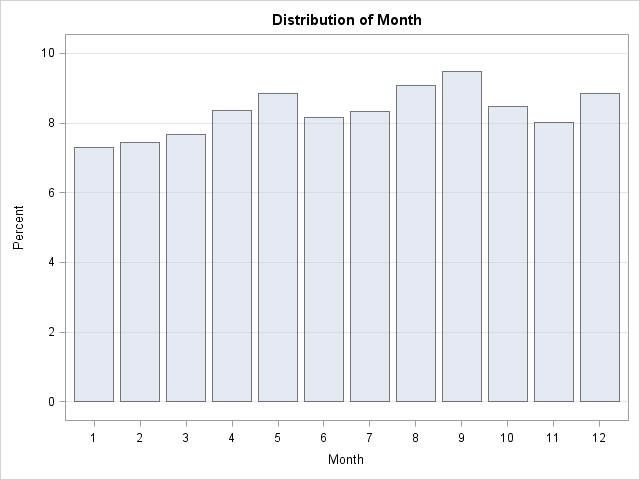The image shows that September birthdays occur most frequently in the sample of 3,061 employees, but it also shows an apparent "bump" in the April–May. The difference between these peak months is small, about 1%, so a random sample with, say, 263 observations can easily contain more spring birthdays than autumn birthdays.

With more work, you can analyze the birthdays by week and fit a periodic smoother to the weekly frequencies of birthdays. This is shown in the following graph: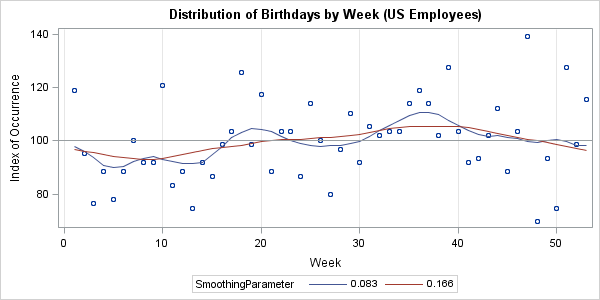The two curves smooth the scatter plot based on a local weighted fit of 12 (blue-gray) or six (red) nearest neighbors. The red curve shows an annual seasonality of fewer births in the winter and more in the late summer and early fall. The blue-gray curve shows that the data might have a smaller cycle with a minor peak near weeks 18–20, in addition to the major peak near weeks 36–38. Both of these curves are consistent with the NCHS report, although the data are not drawn from the same population.

Notice the variability from week to week in this graph. Even with a sample of 3,061, the proportion of birthdays per week varies by ±30%, whereas the 4 million birth records from 2002 show a much smaller week-to-week-variability. For the 2002 data, it was easy to "eyeball" a curve that fit the data. For the birthdays of employees, I would be hard-pressed to predict what a smoothing curve might look like.

If anyone else wants to analyze the 3,061 birthdays, you can download the data as a set of comma-separated values.My elderly mother enjoys playing Scrabble®. The only problem is that my father and most of my siblings won't play with her because she beats them all the time! Consequently, my mother is always excited when I visit because I'll play a few Scrabble games with her.

During a recent visit, I managed to squeak out a couple of rare victories. (The key is to play when she's tired, like right before naptime!) The following panel shows a concise summary of our games (click to enlarge):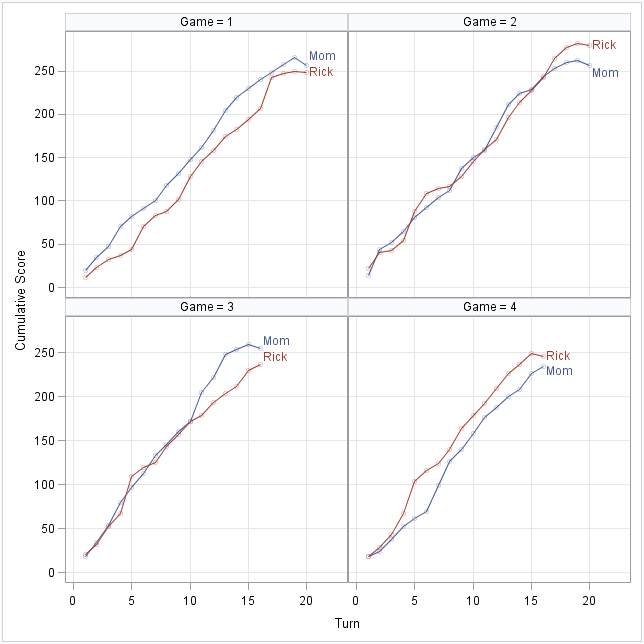The graphic display has the following features:

• You can see the score after each turn.
• You can see the number of turns in each game (16–20).
• The average slope of the line indicates the average number of points per word (about 14 points).
• The gap between the curves shows the difference in scores at each turn.

In particular, you can read off some important plays for each game:

• In Game 1, I was losing the entire game. I almost caught up with a 36-point play at Turn 16, but it was too little, too late.
• In Game 2, the lead changed hands five times, as seen by the crossing of the curves.
• In Game 3, I was holding my own until Mom crushed my hope with a 33-point word on Turn 11.
• In Game 4, I cruised to victory after playing a 37-point word in Turn 4.

I call this a "play-by-play chart." A few years ago, I saw this display used very effectively to show the minute-by-minute evolution of the score of a basketball game, but I can't remember where I saw it.

This display is best used to show the progression of scores for games that feature a lot of scoring, and especially games in which the points per turn can vary. For example, the chart is effective in basketball for seeing the effect of three-point plays and foul shots on the score. This would also be a good display for bowling, golf, and "target shooting" games such as darts and archery. I wouldn't use it for baseball or soccer.

The chart is easy to construct by using the SGPANEL procedure. I added semi-transparent observations in the background, but you can omit the SCATTER statement if you want to see only the lines.

```proc sgpanel data=Scrabble noautolegend; panelby Game; scatter x=Turn y=Cumul / group=Player transparency=0.75; series x=Turn y=Cumul / group=Player curvelabel; rowaxis integer grid; colaxis grid; run;```

If you'd like to try an alternate visualization, you can download the data.

In addition to this play-by-play chart, you might be interested in the distribution of each player's word scores over multiple games. You can use the SGPLOT procedure for each player, or you can combine histograms into a single paneled display by using PROC SGPANEL as follows:

```proc sgpanel data=Scrabble noautolegend; panelby Player; histogram Score; density Score / type=kernel; run;```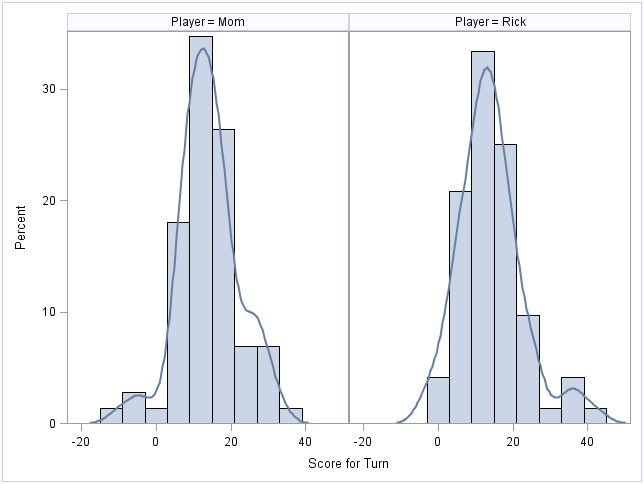The distributions of scores reveal several differences in playing styles and strategies:

• For both players, the typical play scores 12–15 points.
• I tend to use all my tiles by the end of the game. My mother has tiles left over, which results in a penalty as seen by her negative scores at the end of games.
• Both distributions show a "triple word score" peak in the density. Hers is taller than mine is, which indicates that she plays more of the triple word scores. However, my peak is farther to the right, which indicates that my triple word scores tend to be larger. My mother plays a defensive game. If a triple word score space is playable, she grabs it, even if it results in a paltry score for her. (This aggravates my father!)

I only play Scrabble recreationally, but the SGSPANEL procedure enables me to visualize my scores and analyze my playing style. For me, Scrabble is fun to play... and fun to analyze.